# Irodov Solutions: Phase Transformations - 2 Notes | Study I. E. Irodov Solutions for Physics Class 11 & Class 12 - JEE

## JEE: Irodov Solutions: Phase Transformations - 2 Notes | Study I. E. Irodov Solutions for Physics Class 11 & Class 12 - JEE

The document Irodov Solutions: Phase Transformations - 2 Notes | Study I. E. Irodov Solutions for Physics Class 11 & Class 12 - JEE is a part of the JEE Course I. E. Irodov Solutions for Physics Class 11 & Class 12.
All you need of JEE at this link: JEE

Q. 203. What fraction of the volume of a vessel must liquid ether occupy at room temperature in order to pass into critical state when critical temperature is reached? Ether has Tcr = 467 K, pcr  =3 5.5 atm, M = 74 g/mol.

Solution. 203. The vessel is such that either vapour or liquid of mass m occupies it at critical point. Then its volume will be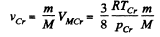The corresponding volume in liquid phase at room temperature is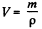where p = density of liquid ether at room tmeperature. Thus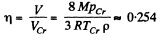using the given data (and p = 720gm per litre)

Q. 204. Demonstrate that the straight line 1-5 corresponding to the isothermal-isobaric phase transition cuts the Van der Waals isotherm so that areas I and II are equal (Fig. 2.5).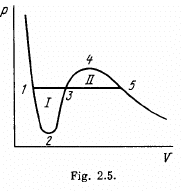Solution. 204. We apply the relation (T = constant)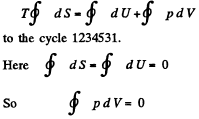This implies that the areas I and II are equal.
This reasoning is inapplicable to the cycle 1231, for example. This cycle is irreversible because it involves the irreversible transition from a single phase to a two-phase state at the point 3.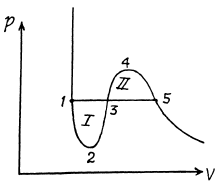Q. 205. What fraction of water supercooled down to the temperature t = —20°C under standard pressure turns into ice when the system passes into the equilibrium state? At what temperature of the supercooled water does it turn into ice completely?

Solution. 205. When a portion of supercool water turns into ice some heat is liberated, which should heat it upto ice point. Neglecting the variation of specific heat of water, the fraction of water turning inot ice is clearly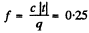where c = specific heat of water and q ≈ latent heat of fusion of ice, Clearly f = 1 at t = -80°C

Q. 206. Find the increment of the ice melting temperature in the vicinity of 0°C when the pressure is increased by Δp = 1.00 atm. The specific volume of ice exceeds that of water by' ΔV' = 0.091 cm3/g.

Solution. 206. From the Claussius-Clapeyron (C-C)equations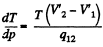q12 is the specific latent heat absorbed in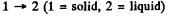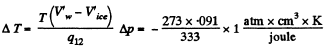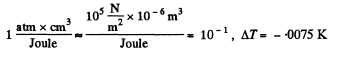Q. 207. Find the specific volume of saturated water vapour under standard pressure if a decrease of pressure by Ap = 3.2 kPa is known to decrease the water boiling temperature by ΔT = 0.9 K.

Solution. 207. Here 1 = liquid, 2 = Steam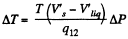or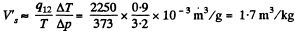Q. 208. Assuming the saturated water vapour to be ideal, find its pressure at the temperature 101.1 °C.

Solution. 208. From C-C equations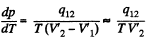Assuming the saturated vapour to be ideal gas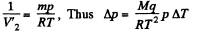and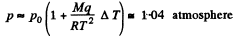Q. 209. A small amount of water and its saturated vapour are enclosed in a vessel at a temperature t = 100°C. How much (in per cent) will the mass of the saturated vapour increase if the temperature of the system goes up by ΔT = 1.5 K? Assume that the vapour is an ideal gas and the specific volume of water is negligible as compared to that of vapour.

Solution. 209. From C-C equation, neglecting the voolume of the liquid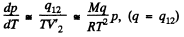or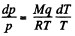Now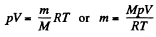for a perfect gas

So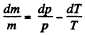(V is Const = specific volume)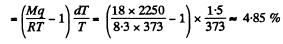Q. 210. Find the pressure of saturated vapour as a function of temperature p (T) if at a temperature T0 its pressure equals p0. Assume that: the specific latent heat of vaporization q is independent of T, the specific volume of liquid is negligible as compared to that of vapour, saturated vapour obeys the equation of state for an ideal gas. Investigate under what conditions these assumptions are permissible.

Solution. 210. From C-C equation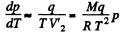Integrating In p = constant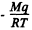So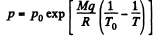This is reasonable for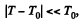and far below critical temperature.

Q. 211. An ice which was initially under standard conditions was compressed up to the pressure p = 640 atm. Assuming the lowering of the ice melting temperature to be a linear function of pressure under the given conditions, find what fraction of the ice melted. The specific volume of water is less than that of ice by ΔV' = 0.09 cm3/g.

Solution. 211. As before (Q.206) the lowering of melting point is given by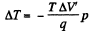The superheated ice will then melt in part. The fraction that will melt is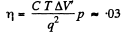Q. 212. In the vicinity of the triple point the saturated vapour pressure p of carbon dioxide depends on temperature T as log p = a — bIT, where a and b are constants. If p is expressed in atmospheres, then for the sublimation process a = 9.05 and b = 1.80 kK, and for the vaporization process a = 6.78 and b = 1.31 kK. Find:
(a) temperature and pressure at the triple point;
(b) the values of the specific latent heats of sublimation, vaporization, and melting in the vicinity of the triple point.

Solution. 212. (a) The equations of the transition lines are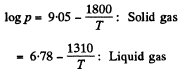At the triple point they intersect Thus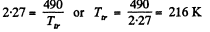corresponding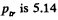atmosphere.

In the formula log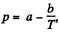we compare b with the corresponding term in the equation in Q.210. Then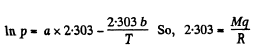or,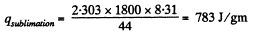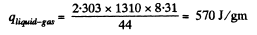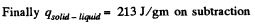Q. 213. Water of mass m = 1.00 kg is heated from the temperature t1 = 10°C up to t2  = 100°C at which it evaporates completely. Find the entropy increment of the system.

Solution. 213.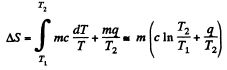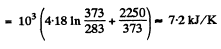Q. 214. The ice with the initial temperature t1 = 0°C was first melted, then heated to the temperature t2 = 100°C and evaporated. Find the increment of the system's specific entropy.

Solution. 214.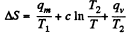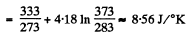Q. 215. A piece of copper of mass m = 90 g at a temperature t1 = 90°C was placed in a calorimeter in which ice of mass 50 g was at a temperature —3°C. Find the entropy increment of the piece of copper by the moment the thermal equilibrium is reached.

Solution. 215. c = specific heat of copper =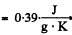Suppose all ice does not melt, then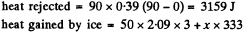Thus    x = 8-5 gm

The hypothesis is correct and final temperature will be T = 273K.

Hence change in entropy of copper piece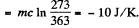Q. 216. A chunk of ice of mass m1 = 100 g at a temperature t1 = 0°C was placed in a calorimeter in which water of mass m2 = 100 g was at a temperature t2. Assuming the heat capacity of the calorimeter to be negligible, find the entropy increment of the system by the moment the thermal equilibrium is reached. Consider two cases: (a) t= 60°C; (b) t2 = 94°C.

Solution. 216. (a) Here t2 = 60°C. Suppose the final temperature is t°C. Then

heat lost by water = m2c (t2 -1)
heat gained by ice = m1qm + m1c (t - t1), if all ice melts

In this case m1 qm = m2 x 4.18 (60 - t)y for m1 = m2

So the final temperature will be 0°C and only some ice will melt.

Then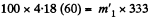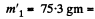amount of ice that will melt

Finally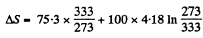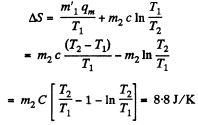(b)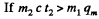then all ice will melt as one can check and the final temperature can be obtained like this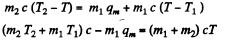or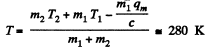and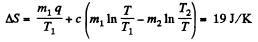Q. 217. Molten lead of mass m = 5.0 g at a temperature t2 = 327°C (the melting temperature of lead) was poured into a calorimeter packed with a large amount of ice at a temperature t1 =  0°C. Find the entropy increment of the system lead-ice by the moment the thermal equilibrium is reached. The specific latent heat of melting of lead is equal to q = 22.5 J/g and its specific heat capacity is equal to c = 0.125 J/(g • K).

Solution. 217.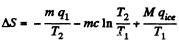where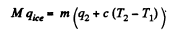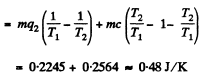Q. 218. A water vapour filling the space under the piston of a cylinder, is compressed (or expanded) so that it remains saturated all the time, being just on the verge of condensation. Find the molar heat capacity C of the vapour in this process as a function of temperature T, assuming the vapour to be an ideal gas and neglecting the specific volume of water in comparison with that of vapour. Calculate C at a temperature t = 100°C.

Solution. 218. When heat dQ is given to the vapour its temperature will change by dT, pressure by dp and volume by dV, it being assumed that the vapour remains saturated.
Then by C-C equation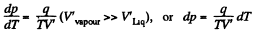on the other hand,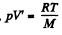So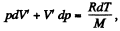Hence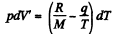finally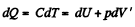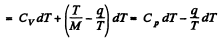(Cp, Cv refer to unit mass here). Thus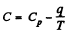For water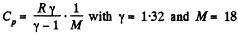So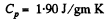and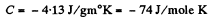Q. 219. One mole of water being in equilibrium with a negligible amount of its saturated vapour at a temperature T1 was completely converted into saturated vapour at a temperature T2. Find the entropy increment of the system. The vapour is assumed to be an ideal gas, the specific volume of the liquid is negligible in comparison with that of the vapour.

Solution. 219. The required entropy change can be calculated along a process in which the water is heated from T1 to T2 and then allowed to evaporate. The entropy change for this is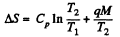where q = specific latent heat of vaporization.

The document Irodov Solutions: Phase Transformations - 2 Notes | Study I. E. Irodov Solutions for Physics Class 11 & Class 12 - JEE is a part of the JEE Course I. E. Irodov Solutions for Physics Class 11 & Class 12.
All you need of JEE at this link: JEEUse Code STAYHOME200 and get INR 200 additional OFF

## I. E. Irodov Solutions for Physics Class 11 & Class 12

111 docs

Track your progress, build streaks, highlight & save important lessons and more!

,

,

,

,

,

,

,

,

,

,

,

,

,

,

,

,

,

,

,

,

,

;